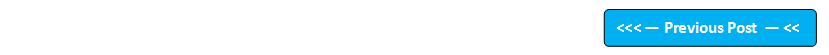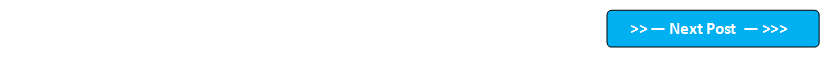Google+

## Blog Pages

### 172. Using 'length' array attribute with 2 dimensional arraysPre-requisites -

1. Post # 48 Arrays
2. Post # 92 'length' array attribute

Assuming that you have understood the array concepts from the above pre-requisite posts, I would like to explain how to use 'length' attribute to calculate the number of rows and number of columns in a 2 dimensional array.

Example of a 2 dimensional array -

int a[][] = new int;

Visual representation of the array -

To calculate the number of rows in this array we have to use -> a.length

To calculate the number of columns in first row of the array we have to use -> a.length

To calculate the number of columns in second row of the array we have to use -> a.length

In a 2D array, which is having the same number of columns in every row will give the same result for a.length and a.length

Lets implement this on Eclipse IDE -

1. Launch Eclipse IDE, create a new Java Project 'Project 018' as shown below -

2. Create a Java Class 'Class018' with main( ) method as shown below -

3. Declare a two dimensional array as shown below -

4. Using length attribute to calculate the number of rows and storing the result in the integer variable 'rows' as shown below -

5. Using length attribute to calculate the number of columns in the first row of the array and storing the result in the integer variable 'row1_columns' as shown below -

6. Using length attribute to calculate the number of columns in the second row of the array and storing the result in the integer varaible 'row2_columns' as shown below -

7. Print the calculated number of rows, number of columns in first and second rows as shown below -

8. Run the Java Class and observe that the following output is displayed under the Console as shown below -

This is how, we use the length attribute in a two dimensional array.

Using length attribute in a three dimensional array will be explained in the next post.

Please comment below to feedback or ask questions.

Using 'length' attribute with 3 dimensional arrays  will be explained in the next post.